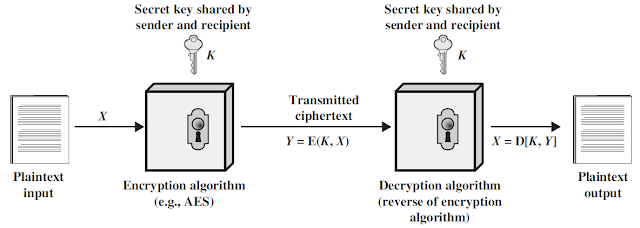## Thursday, September 23, 2021

### Symmetric Key Cryptography | Secret Key Cryptography

Symmetric Key Cryptography

Same key is used for encryption and decryption is called symmetric key cryptography. It is also known as secret key cryptography.

Elements of Symmetric Key Cryptography

Plaintext is the original message or data that is fed into the algorithm as input.

Encryption algorithm performs various substitutions and transformations on the plaintext.

Secret key is a value independent of the plaintext and of the algorithm. The exact substitutions and transformations performed by the algorithm depend on the key.

Ciphertext is the unreadable message produced as output. It depends on the plain text and secret key.

Decryption algorithm takes the cipher text and the secret key and produces the original plain text.

Same key is used for encryption and decryption process. So, it is called symmetric cipher.Figure: Symmetric Key Cryptography

Mathematically it is represented as

For Encryption, Y = E (K, X)

For Decryption, X = D (K, Y)

where Y = Cipher text, X = Plain Text, E = encryption, D = Decryption, K = Secret shared key.

For example, Data Encryption Standards (DES), Advanced Encryption Standards (AES) and BLOWFISH.